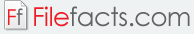### Curvefit 10.1

#### Decision Support Systems (Bundled)

Curve Fit is a pixel level raster regression tool developed by Decision Support Systems. The program is an ArcMap extension. ArcMap is a GIS application. The program enables users to run regression analysis functions for raster datasets. An explanatory variable or X is rendered from an array of values entered by the user. Each X value entered by the user is paired with a Y value supplied by the program, thus creating a raster dataset.  Then, the program utilizes a nonlinear or a linear regression technique which the user can predetermine. The program then analyzes the input raster datasets and calculates a unique mathematical model for each pixel.

Curve Fit also renders raster surfaces of error, parameter estimates, and multi-model inference. The program is a predictive tool, as well as an explanatory tool. The predictive function enables spatial modelers to perform key statistical functions. The program features both nonlinear and linear raster regression techniques. For the nonlinear functions, users can configure the program to manipulate parameters, tolerances, and solution iterations. In creating linear regression models, users have the option to utilize either linear or polynomial functions. When in nonlinear regression mode, users has three options—Power, Four Parameter Logistic, and Exponential.

Curve Fit has several output product scopes. These are parameter, model, and data point. Raster products describe parameter estimate, error estimate, goodness of fit, and multi-model inference.